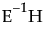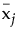where Y is the matrix of response variables, M is the response design matrix, and V is the matrix of eigenvectors offor the given test. Canonical Y’s are saved for eigenvectors corresponding to eigenvalues larger than zero.
where V is the matrix of eigenvectors of.
and the vs are columns of V, the eigenvector matrix of,refers to the multivariate least squares mean for the jth effect, g is the number of eigenvalues ofgreater than 0, and r is the rank of the X matrix.
where g is the number of eigenvalues ofgreater than 0 and the denominator L’s are from the multivariate least squares means calculations.

Help created on 9/19/2017